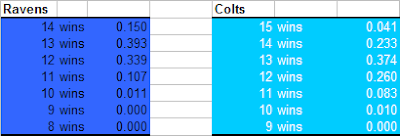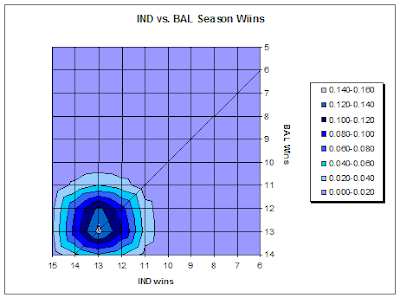## Predicting Playoff Races

Building upon the Total Probabilities calculations I did beginning after week 12 which forcast the probabilities of a team to finish with a total number of wins, I could then compare multiple teams competing for playoff spots.

I'll use Baltimore as an example again, because I follow the Ravens most closely. Following week 12, the Ravens were 9-2 and it was all but certain they would win the AFC North. But they were also in the hunt for a first-round bye in the playoffs. Indianapolis held the second seed and a bye with a 10-1 record. So to earn a bye, BAL had to at least beat out IND.

Since the model had calculated, based on a game-by-game assessment, the probabilities of each team finishing the season with each possible total number of wins, they could be compared side-by-side. Here is how both teams' outlooks appeared following week 12.The probability of every possible combination of outcomes can be calculated by multiplying the individual component probabilities. For example, the probability of BAL and IND both finishing the season with 12 wins would be:

Prob(BAL 12, IND 12) = Prob(BAL 12) * Prob(IND 12) = .339 * .260 = .088

So there is about a 9% chance of that particular outcome. Every possible combination of outcomes is listed in the table below. BAL win totals are listed vertically on the left and IND win totals are listed horizontally on top. (Click to enlarge.)The outcomes shaded in gray are ties, where both teams finish with equal numbers of wins. In this case BAL held the tie-breaker (better record vs. common opponents). The outcomes in green are those for which BAL finishes with more wins, and the outcomes in red are those for which IND finishes with more wins. It's quite a jumble of numbers, but there are really only 3 possible outcomes that matter: BAL wins, IND wins, or they tie. We can simply sum up all the green, red, and grey outcomes to finally produce the probabilities of each outcome.

P(BAL wins) = .279
P(Tie) = .278
P(IND wins) = .443

(Total = 1.000)

The table above is easier to understand if pictured graphically. Below is a plot of the probabilities of each outcome. BAL's wins are along the vertical axis and IND's are along the horizontal axis. The diagonal line plots the tie outcomes, where both teams finish with an equal number of wins. Plot "density" above the line indicates IND would have more wins, and "density" below the line indicates BAL would have more wins. Here, we see the "center of gravity" of the probability plot shows the mostly likely outcome is a tie at 13 wins for both teams. (And except for IND laying an egg at home vs. HOU, this would have been the real outcome.)BAL actually seemed to have an advantage, because they owned the tie-breaker, and in fact, BAL ended the season 1 game ahead of IND to capture the second seed and a bye in the playoffs.

You can download the spreadsheet with several selected match-ups between playoff contenders here.

### 1 Responses to “Predicting Playoff Races”

1. j-mo says:

Wow. The Ravens are awesome, aren't they?

### Leave a Reply

Note: Only a member of this blog may post a comment.